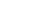You've successfully subscribed to The Finbox Blog
Welcome back! You've successfully signed in.# Dividend Payout Ratio Explained

Investors are always looking for high dividend yield stocks that can (in theory) provide them with a high return on their investment. However, as you learned in a previous lesson of the dividend investing course, stocks with the highest dividend yields tend to underperform those with lower yields because higher yields often reflect higher underlying risk.

When a company's dividend yield is abnormally high, the market may be expecting management to announce a dividend cut. In this lesson, you will learn how to use the dividend payout ratio to spot a risky dividend before it's too late and determine if a company's distributions to shareholders are sustainable in the long term.

#### What Is The Dividend Payout Ratio?

If you are an income investor, and you want to pick only the best dividend stocks, the dividend payout ratio can make the difference between great and bad investment decisions.

For example, if two stocks have the same dividend yield and are nearly equal in other respects, you want to pick the one with the lower dividend payout ratio. A lower dividend payout ratio suggests that the company retains a larger percentage of earnings to reinvest and grow while paying you the same amount in the form of dividends.

### How To Calculate The Dividend Payout Ratio With The Right Formula?

As previously mentioned, the dividend payout ratio is equal to the total dividends paid to shareholders divided by the net income.

Dividend Payout Ratio = Total Dividends / Net income

You can also calculate it by dividing the dividend per share by the earnings per share. Here's the formula:

Dividend Payout Ratio = Dividends Per Share / EPS

The dividend per share is equal to the total dividends paid by the company, divided by the number of ordinary shares outstanding.

The earnings per share is a financial metric equal to the net profit divided by the number of ordinary shares outstanding.## Dividend Payout Ratio Example Calculation

We can use the formula mentioned earlier to calculate Microsoft's  (NASDAQGS:MSFT) dividend payout ratio, as follows:

Microsoft Dividend Payout Ratio = Microsoft Total Dividends / Microsoft Net Income

Using the Finbox data explorer, we can find the values of the metrics required for the calculation:

• Microsoft's Total Dividends: \$14.793B
• Microsoft's Net Income: \$46.266B

We can now calculate Microsoft dividend payout ratio by dividing the total dividends by the net income:

Microsoft Dividend Payout Ratio = \$14.793 / \$46.266 = 32%

That means that Microsoft distributes 32% of its earnings to shareholders while retaining the rest to invest and grow the business.

Pro Tip: You don't need to do the calculation alone and you don't have to use a dividend payout ratio calculator. You can use the Finbox Data Explorer to access dividend payout ratio data for 100,000+ companies worldwide.

### Dividend Payout Ratio: Sector Benchmarks

As of September 8, 2020, the sectors with the highest dividend payout ratio are real estate, utilities, and consumer staples. Communication services, consumer discretionary, and Healthcare are the sectors with the lowest dividend payout ratio.Pro Tip: You can get up to date stats and graphs like that directly in Excel/Google Sheets using this model following the instructions inside.

### What Is A Good Dividend Payout Ratio?

If you try searching for an appropriate dividend payout ratio on Google, you will find a wide range of answers. For example, some resources state that a good dividend payout ratio is below 30%, others say that a healthy payout ratio is between 30% and 50%, and so on.

However, one way quick to determine a healthy payout ratio is by comparing it to the sector average. As you can see in the picture above, the dividend payout ratio varies considerably from one sector to another, ranging from 114% for real estate to 27% for industrials.

As a rule of thumb, a good dividend payout ratio is one that is in line with the sector average. When a company pays a dividend that is high relative to its earnings and/or compared to others in the same sector, there is a strong chance that the distribution is not sustainable in the long term.

Warning: Investing in businesses with a too high dividend payout ratio could cost you a lot of money. You can check if the dividend payout ratio of your companies is sustainable in the long term by comparing it to the sector average using the Finbox Data Explorer.

Tags